### Vector Equation of the Plane

To determine the equation of a plane in 3D space, a point P and a pair of vectors which form a basis (linearly independent vectors) must be known.The point P belongs to the plane π if the vectoris coplanar with the vectorsand.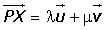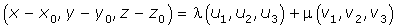### Parametric Equations of the Plane### Cartesian Equation of the Plane

A point is in the plane π if the system has the solution:The values are given as:### Intercept FormA(a, 0, 0), B(0, b, 0) and C(0, 0, c).### Examples

1.Find the equations of the plane that pass through point A = (1, 1, 1) and their direction vectors are: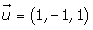and.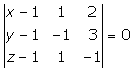2.Find the equations of the plane that pass through points A = (−1, 2, 3) and B = (3, 1, 4) and contains the vector.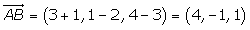3.Find the equations of the plane that pass through points A = (−1, 1, −1), B = (0, 1, 1) and C = (4, −3, 2).4. π is the plane of parametric equations:Confirm whether the points A = (2, 1, 9/2) and B = (0, 9, −1) belong to this plane.5.Find the equation of the plane in intercept form that passes through the points A = (1, 1, 0), B = (1, 0, 1) and C = (0, 1, 1).Divide by −2, and the equation is obtained:6.Find the equation of the plane that passes through the point A = (2, 0, 1) and contains the line with the equation:From the equation of the line, a second point and the vector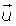is obtained.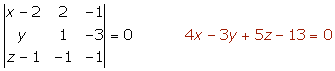7.Find the equation of the plane that passes through the points A = (1, −2, 4) and B = (0, 3, 2) and is parallel to the line:8.Given the lines:Determine the equation of the plane that contains the line r and is parallel to the line s.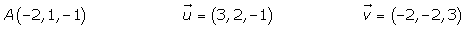Did you like the article?(1 votes, average: 5.00 out of 5)Loading...

Emma

I am passionate about travelling and currently live and work in Paris. I like to spend my time reading, gardening, running, learning languages and exploring new places.

Did you like
this resource?

Bravo!